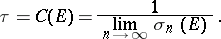# Chebyshev constant

Jump to: navigation, search

A numerical invariant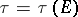of a compact setin the complex plane that is used in the theory of best approximation.

Letbe the class of all polynomials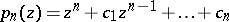of degree, and let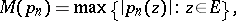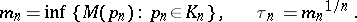There exists a polynomialfor which; it is called the Chebyshev polynomial for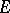. Moreover, the limit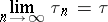exists, and is called the Chebyshev constant for.

Restricting oneself to the classof all polynomials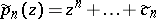all zeros of which lie in, one obtains corresponding values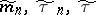and a polynomial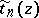for which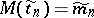(it is also called the Chebyshev polynomial).

It is known that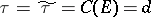, where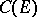is the capacity of the compact set, andis its transfinite diameter (cf., for example, ).

The concept of the Chebyshev constant generalizes to compact setsin higher-dimensional Euclidean spaces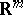starting from potential theory. For a point, let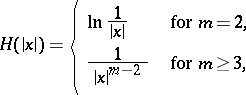be the fundamental solution of the Laplace equation, and for a set, let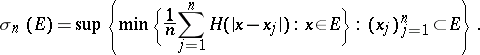Then forone obtains the relationand for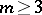one obtains (cf. ):